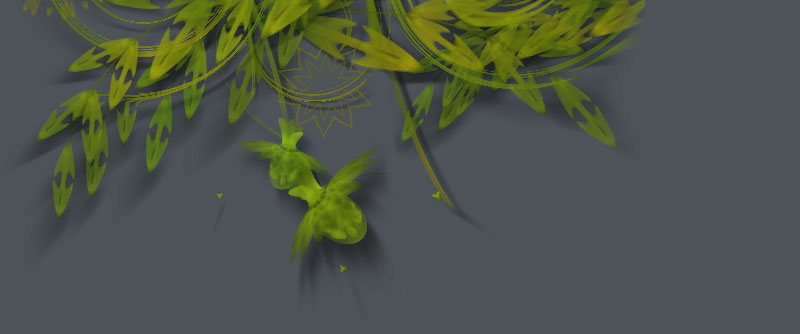### Compound paths

Paths in NodeBox support so-called boolean operations. This means you can create a new compound path from a combination of other paths. This is useful if you want to create a shape from a combination of other shapes (like ovals and rectangles) and then flatten the group into a single path. Manipulating a single shape is much easier and faster than manipulating a group of shapes.

`path1.union(path2, flatness=0.6)`
`path1.intersect(path2, flatness=0.6)`
`path1.difference(path2, flatness=0.6)`

Each of the three methods returns a new path combined from the two given paths. The way in which the new path is traced differs for each method, as you can observe below:Union traces and merges the two paths, removing any overlapping parts. ```nofill() stroke(0) path1 = oval(40, 40, 80, 80, draw=False) path2 = oval(90, 40, 80, 80, draw=False) compound = path1.union(path2) drawpath(compound)```Intersect traces the overlapping region and removes everything else.```nofill() stroke(0) path1 = oval(40, 40, 80, 80, draw=False) path2 = oval(90, 40, 80, 80, draw=False) compound = path1.intersect(path2) drawpath(compound)```Difference subtracts the second shape from the first shape.```nofill() stroke(0) path1 = oval(40, 40, 80, 80, draw=False) path2 = oval(90, 40, 80, 80, draw=False) compound = path1.difference(path2) drawpath(compound)```XOR traces non-overlapping regions, excluding any areas that overlap.```fill(0.8) stroke(0) path1 = oval(40, 40, 80, 80, draw=False) path2 = oval(90, 40, 80, 80, draw=False) compound = path1.xor(path2) drawpath(compound)```

The returned path can be manipulated like any other path in NodeBox, you can fill() and stroke() it, rotate() it and put it (or multiple copies of it) onscreen with drawpath().

One thing to note is that the compound path will not contain any curves, rather, it is made up of numerous straight line segments (we say that the path is flattened). Each of the compound methods has an optional flatness parameter. The lower it gets, the more line segments will be drawn (and hence the smoother the resulting shape).

## Flattening stack

With a for-loop we can keep adding new paths to the compound path. In the example below, we create a number of circles varying in size, and append them to one flattened compound path:

```compound = None
for i in range(50):
r = random(75)
path = oval(random(300), random(200), r, r, draw=False)
if not compound:
compound = path
compound = compound.union(path)

nofill()
stroke(0)
drawpath(compound)```## Using a Transform object

It's easy enough to draw transformed and colored copies of the compound path, but what if we want to rotate and scale the individual shapes before flattening them? We can use the Transform object in NodeBox for this purpose.

```# We construct our indivual shape in the top-left corner.
# This is where corner-mode transforms originate.
# We'll later on place the eventual compound path
# where we want it with translate().
path = rect(-10, 0, 20, 100, draw=False)

# Let's chip away small piece of the rectangle:
path = path.difference(rect(-15, 80, 20, 15, draw=False))

compound = path
for i in range(17):

# Before adding the rectangle shape to
# the flattened compound, we rotate it a bit.
t = Transform()
t.rotate(20)
path = t.transformBezierPath(path)

compound = compound.union(path)

translate(275, 275)
nofill()
stroke(0)
drawpath(compound)```